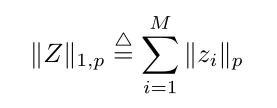# The CVX problem of expressing row-based matrix l2-normalization

Hello, guys.
A very intractable problem confused me. I can’t deal with that. Please help me .I’m striggle with it for one day.
I need kind guys to teach me how to do.
Here is the problem：
I just want to sovle a non-limited minimal optimal problem with CVX.
In the objective function, there is a term of row-based l2-norm of matrix Z.
n times n Matrix Z is a variable and is typoed as variables Z(n,n)where, p is p-norm, z_i is the rows of the matrix Z
Thanks very much for your kind help.

`sum(norms(Z,p,2))` does what you want.

help norms

norms Computation of multiple vector norms.
norms( X ) provides a means to compute the norms of multiple vectors
packed into a matrix or N-D array. This is useful for performing
max-of-norms or sum-of-norms calculations.

``````All of the vector norms, including the false "-inf" norm, supported
by NORM() have been implemented in the norms() command.
norms(X,P)           = sum(abs(X).^P).^(1/P)
norms(X)             = norms(X,2).
norms(X,inf)         = max(abs(X)).
norms(X,-inf)        = min(abs(X)).
If X is a vector, these computations are completely identical to
their NORM equivalents. If X is a matrix, a row vector is returned
of the norms of each column of X. If X is an N-D matrix, the norms
are computed along the first non-singleton dimension.

norms( X, [], DIM ) or norms( X, 2, DIM ) computes Euclidean norms
along the dimension DIM. norms( X, P, DIM ) computes its norms
along the dimension DIM.

Disciplined convex programming information:
norms is convex, except when P<1, so an error will result if these
non-convex "norms" are used within CVX expressions. norms is
nonmonotonic, so its input must be affine.``````
1 Like